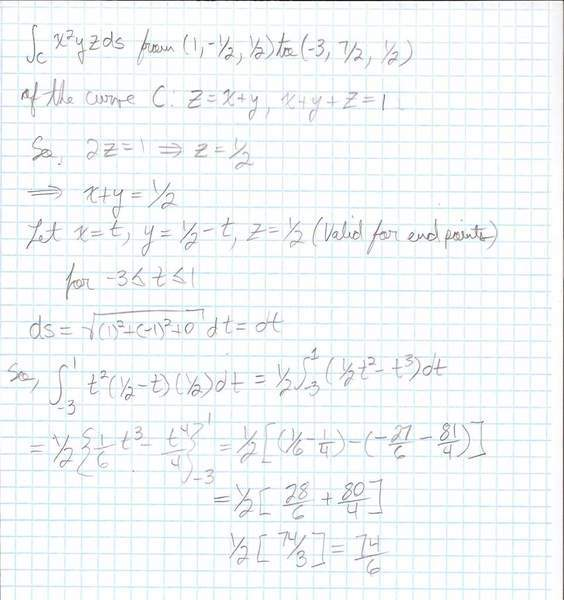# Simple Line Integral Problem

## Homework Statement

Evaluate the line integral,

$$\int_{C}x^{2}yzds, \text{ where C is the curve } z = x + y, \quad x + y + z = 1\text{ from }(1,\frac{-1}{2},\frac{1}{2}) \text{ to } (-3, \frac{7}{2}, \frac{1}{2})$$

## The Attempt at a Solution

Here's my attempt at the problem,It lists the correct answer as, $$\frac{37\sqrt{2}}{3}$$ solved with different parametric equations and bounds for t as well.

What am I doing wrong?

#### Attachments

$$\sqrt{(1)^{2}+(-1)^{2}} \ne 1$$